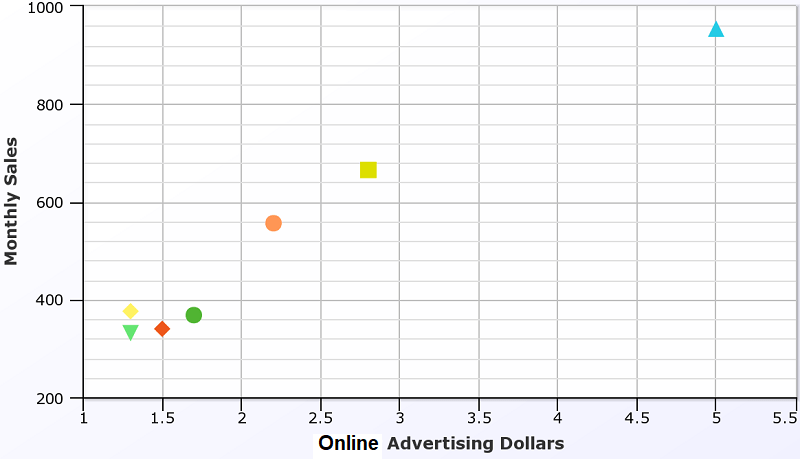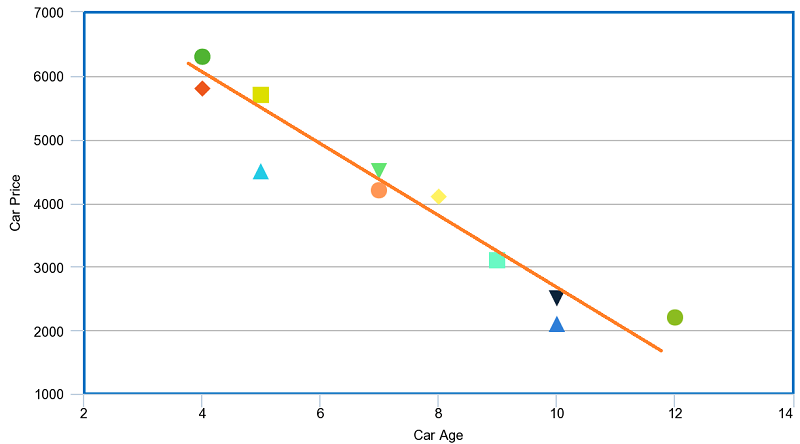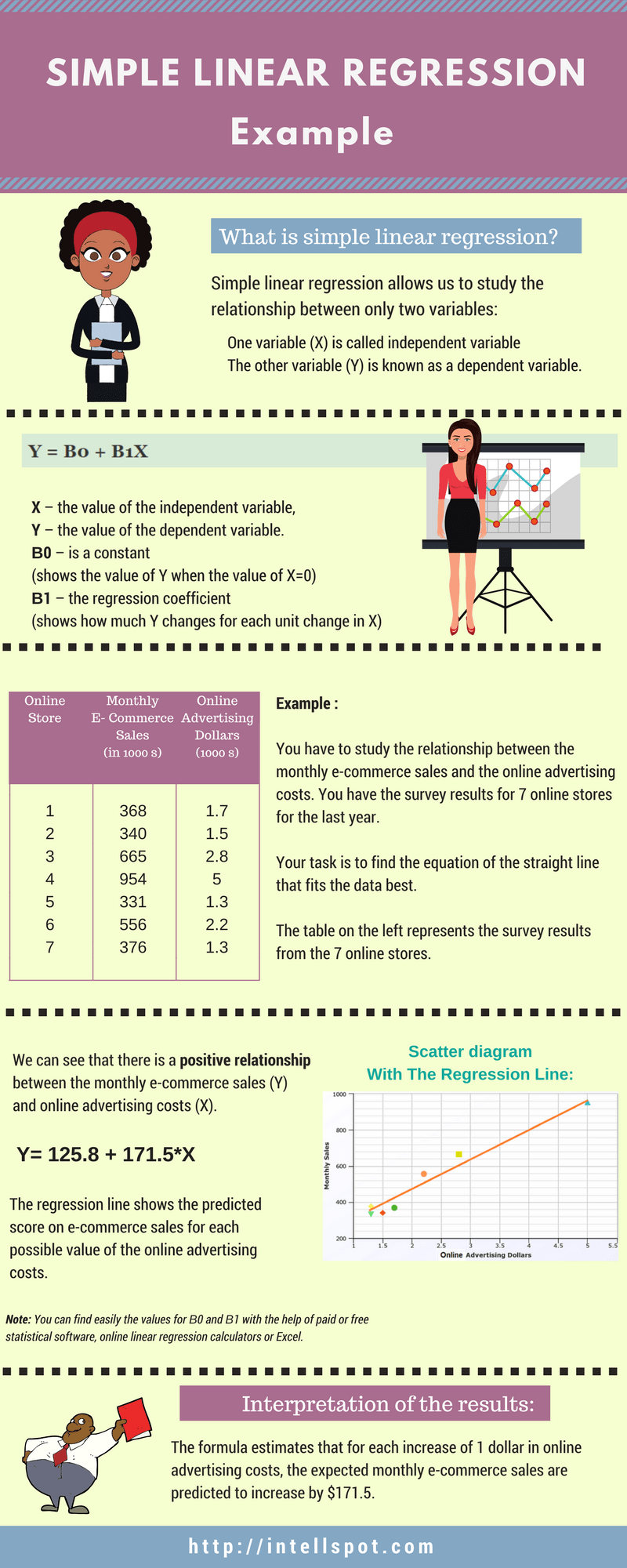Simple Linear Regression Examples

Many of simple linear regression examples (problems and solutions) from the real life can be given to help you understand the core meaning.

From a marketing or statistical research to data analysis, linear regression model have an important role in the business.

As the simple linear regression equation explains a correlation between 2 variables (one independent and one dependent variable), it is a basis for many analyses and predictions. Apart from the business, LR is used in many other areas such as analyzing data sets in statistics, biology or machine learning projects and etc.

• Simple linear regression examples: problems with solutions.
• Infographic in PDF

In our previous post linear regression models, we explained in details what is simple and multiple linear regression. Here, we concentrate on the examples of linear regression from the real life.

Simple Linear Regression Examples, Problems, and Solutions

Let's remind that:

Simple linear regression allows us to study the correlation between only two variables:

• One variable (X) is called independent variable or predictor.
• The other variable (Y), is known as dependent variable or outcome.

and the simple linear regression equation is:

Y = Β0 + Β1X

Where:

X – the value of the independent variable,
Y – the value of the dependent variable.
Β0 – is a constant (shows the value of Y when the value of X=0)
Β1 – the regression coefficient (shows how much Y changes for each unit change in X)

Example 1:

You have to study the relationship between the monthly e-commerce sales and the online advertising costs. You have the survey results for 7 online stores for the last year.

Your task is to find the equation of the straight line that fits the data best.

The following table represents the survey results from the 7 online stores.

 Online Store Monthly E-commerce Sales (in 1000 s) Online Advertising Dollars (1000 s) 1 368 1.7 2 340 1.5 3 665 2.8 4 954 5 5 331 1.3 6 556 2.2 7 376 1.3

We can see that there is a positive relationship between the monthly e-commerce sales (Y) and online advertising costs (X).

The positive correlation means that the values of the dependent variable (y) increase when the values of the independent variable (x) rise.

So, if we want to predict the monthly e-commerce sales from the online advertising costs, the higher the value of advertising costs, the higher our prediction of sales.

We will use the above data to build our Scatter diagram.

Now, let’ see how the Scatter diagram looks like:

Diagram 1:The Scatter plot shows how much one variable affects another. In our example, above Scatter plot shows how much online advertising costs affect the monthly e-commerce sales. It shows their correlation.

Let’s see the simple linear regression equation.

Y = Β0 + Β1X

Y= 125.8 + 171.5*X

Note: You can find easily the values for Β0 and Β1 with the help of paid or free statistical software, online linear regression calculators or Excel. All you need are the values for the independent (x) and dependent (y) variables (as those in the above table).

Now, we have to see our regression line:

Graph of the Regression Line:

Diagram 2Linear regression aims to find the best-fitting straight line through the points. The best-fitting line is known as the regression line.

If data points are closer when plotted to making a straight line, it means the correlation between the two variables is higher. In our example, the relationship is strong.

The orange diagonal line in diagram 2 is the regression line and shows the predicted score on e-commerce sales for each possible value of the online advertising costs.

Interpretation of the results:

The slope of 171.5 shows that each increase of one unit in X, we predict the average of Y to increase by an estimated 171.5 units.

The formula estimates that for each increase of 1 dollar in online advertising costs, the expected monthly e-commerce sales are predicted to increase by \$171.5.

This was a simple linear regression example for a positive relationship in business. Let’s see an example of the negative relationship.

Example 2:

You have to examine the relationship between the age and price for used cars sold in the last year by a car dealership company.

Here is the table of the data:

 Car Age (in years) Price (in dollars) 4 6300 4 5800 5 5700 5 4500 7 4500 7 4200 8 4100 9 3100 10 2100 11 2500 12 2200

Now, we see that we have a negative relationship between the car price (Y) and car age(X) – as car age increases, price decreases.

When we use the simple linear regression equation, we have the following results:

Y = Β0 + Β1X

Y = 7836 – 502.4*X

Let’s use the data from the table and create our Scatter plot and linear regression line:

Diagram 3:The above 3 diagrams are made with Meta Chart.

Result Interpretation:

With an estimated slope of – 502.4, we can conclude that the average car price decreases \$502.2 for each year a car increases in age.

The above simple linear regression examples and problems aim to help you understand better the whole idea behind simple linear regression equation.

Problem-solving using linear regression has so many applications in business, social, biological, and many many other areas.

If you need more examples in the field of statistics and data analysis, our posts “descriptive statistics examples” and “binomial distribution examples” might be useful to you.

Download the following infographic in PDF with the simple linear regression examples: